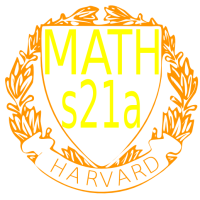Course 30189: Maths21A Summer 2008
Multivariable Calculus
Exams
Office: SciCtr 434

 Final exam: took place on 8/12 8:30 in Emerson 101 The final exam had an average score of 72 with a standard deviation of 17. Exams are still being double checked in the moment. These are preliminary statistics.

2. Midterm:Thu 7/249:30-11Usual Lecture Hall
The second midterm had an average score of 78, with a standard deviation 16.

 1. Midterm: Thu 7/10 9:30-11 Usual Lecture Hall The first midterm had an average score of 76, with a standard deviation 15. Errata: Practice exam 3: TF problem 18 was labeled incorrectly. It is false as the explanation tells. (Thanks to Aukosh) Practice exam 3: TF problem 16 was labeled wrong because it was not z=g(x,y). It is not a good problem. If you have z = g(x,y), then the parametrization is r(u,v) = (u,v,g(u,v)). The problem is now changed. (Thanks for the alert by Aukosh). Practice exam 1: sotution to one matching problem was not complete (Thanks to Chinmay Paranjape). Practice exam 2: 4) solution was missing, 6d) used wrong data. Improvements: parametrization of torus uses 2+sin(phi). (Thanks to Wenying Huang). Practice exam 3: Problem 10 uses point (5,0,2) not (5,2,0) (Thanks to Wenying Huang).